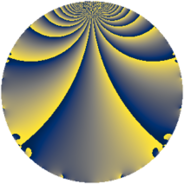# Properties

 Label 1205.2.kLevel $1205$ Weight $2$ Character orbit 1205.k Rep. character $\chi_{1205}(64,\cdot)$ Character field $\Q(\zeta_{4})$ Dimension $240$ Sturm bound $242$

# Related objects

## Defining parameters

 Level: $$N$$ $$=$$ $$1205 = 5 \cdot 241$$ Weight: $$k$$ $$=$$ $$2$$ Character orbit: $$[\chi]$$ $$=$$ 1205.k (of order $$4$$ and degree $$2$$) Character conductor: $$\operatorname{cond}(\chi)$$ $$=$$ $$1205$$ Character field: $$\Q(i)$$ Sturm bound: $$242$$

## Dimensions

The following table gives the dimensions of various subspaces of $$M_{2}(1205, [\chi])$$.

Total New Old
Modular forms 248 248 0
Cusp forms 240 240 0
Eisenstein series 8 8 0

## Trace form

 $$240q + 240q^{4} - 32q^{6} + 240q^{9} + O(q^{10})$$ $$240q + 240q^{4} - 32q^{6} + 240q^{9} - 20q^{10} - 4q^{14} - 16q^{15} + 224q^{16} - 8q^{19} - 12q^{21} - 104q^{24} + 24q^{25} + 12q^{26} - 4q^{31} - 32q^{34} - 2q^{35} + 224q^{36} - 44q^{40} - 44q^{44} + 16q^{46} - 176q^{54} + 34q^{55} - 32q^{56} - 92q^{60} + 232q^{64} - 32q^{65} + 76q^{66} - 20q^{69} + 24q^{70} - 36q^{71} - 68q^{74} - 112q^{76} + 176q^{81} - 128q^{84} - 26q^{85} + 12q^{86} - 180q^{90} + 16q^{91} - 120q^{94} - 44q^{95} - 104q^{96} - 24q^{99} + O(q^{100})$$

## Decomposition of $$S_{2}^{\mathrm{new}}(1205, [\chi])$$ into newform subspaces

The newforms in this space have not yet been added to the LMFDB.